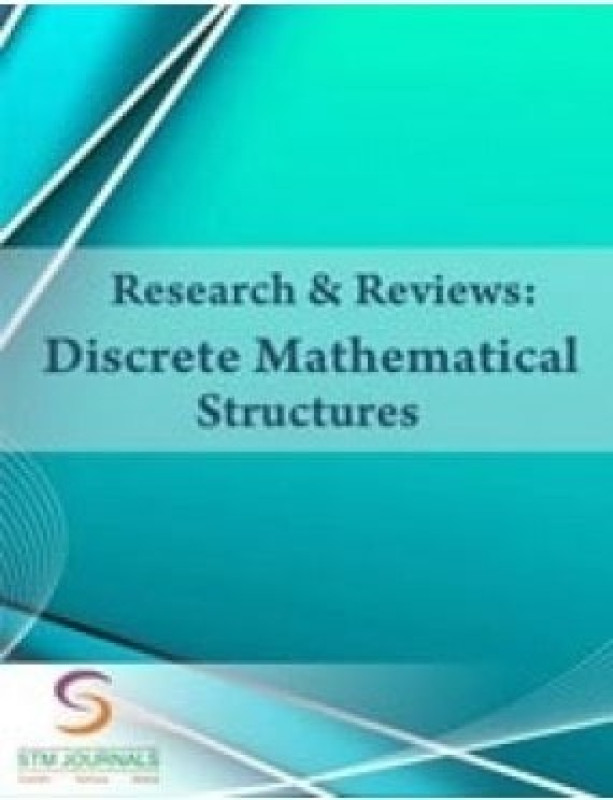The Week Magazine Offer
New Prize With 1 Year Subscription

# Research and Reviews Discrete Mathematical Structures• Publisher: Consortium eLearning Network Private Limited
• Language: English
• Frequency of Publication: Tri yearly
Subscription Cover Price (₹) Offer Price (₹) Issues Gift
1 Year 3950.00 3950.00 0% Off 2 Issues NA
1 Year Print + 1 Year Digital 8880.00 8880.00 0% Off 2 Issues NA
1 Year Digitial 7900.00 7900.00 0% Off 2 Issues NA

Research & Reviews: Discrete Mathematical Structures (RRDMS): Discrete mathematical structures journal deals with discrete objects. Discrete objects are those which are separated from each other like integers, rational numbers, automobiles, houses, peoples etc. are all discrete objects. Some of the major reasons that we adopt discrete mathematics are. We can handle infinity or large quantity and indefiniteness with them which results from formal approaches are reusable.

Focus and Scope Covers

• Mathematical induction
• Logic and boolean algebra
• Set theory
• Relations and functions
• Sequences and series
• Algorithms and theory of computation
• Number theory
• Matrix theory
• Induction and recursion
• Counting and discrete probability
• Graph theory (trees)
• Calculus of finite differences, discrete calculus or discrete analysis
• Game theory, decision theory, utility theory, social choice theory
• Discrete analogues of continuous mathematics
• Hybrid discrete and continuous mathematics

Note: Delivery in 4-6 weeks.